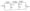# CSVTU Basic Electrical Engineering Question Paper Dec 2007

## Basic Electrical Engineering

Q1 (a)  Define Kirchhoffs current and voltage law .

(b) Solve the network given for the following

(i) Unknown resistances R1 and R2

(ii)  Unknown currents in various branches of the circuit.(c) Find the current through the 20 resistor using.The venin’s theorem(d) A cast steel ring has circular cross section 3 cm diameter  mean circumference of 80cm . The ring is uniformly would coil of 600 turns.

(e) Estimate the current required to produce a flux of 0.5 pub through the ring

(i) If a saw cut 2mm wide is made , find approximately frie fk produced by the current in (i)

(ii) Find the current value which will give the same flux as in 1 neglect fringing and leakage and =800

Q2 (a) Explain active, reactive and apparent power with pow triangle.

(b) Find the vases of R and C so that  in quadrate Find also the phase relation between V and I.(c)Two impedances z1 and z2 are connected in parallel the fir branches takes a leading current of 16 a and has resistance of 5 while the second branch takes a leading current at power factor 0.8 the total power supplied is 5 kV the applied voltage being (100+ j200)V . Determine the complex expression for the branch and total currents and  the circuit constants.

(d) For the measurement of power in a three phase , star connected load using 2 wattmeter method. In such an experiment the load supplied was 30 kiwi at 0.7 of lagging. Find the reading of such wattmeter . Foe what pf. Will one of the wattmeter read zero.

Q3(a)  Derive the elf equation of a single phase transforms.

(b) Draw the pharos diagram of a single phase transformer at no load for a inductive load neglecting the winding resistances and leakage reactance’s.

(c)  The following results were obtained on a 50kVA , 2400/120V transformer

O.C. test: instrument on 1v side

Voltmeter reading = 12V S.C. test: instruments on h.v side

A Voltmeter reading = 92 V

Determine

(i) the circuit constants

(ii)the efficiency at full load 0.8 puff, lagging.

(iii)the approximate voltage regulation.

(b)A 100 kava, 50Hz, 440/11000V, Single phase transformer has an efficiency of 98.5% when supplying foil load current at 0.8 puff, lagging and an efficiency of 99% when supplying half full load current at unity power factor. Find the core losses and Cu losses corresponding of a dc machines

Q4 (a) A3-phase 6 pole, 50Hz induction motor has a slip of 1 % at no load and 3% at full load. Determine

(i) synchronous speed :

(iii) frequency of rotor current at stand still,

(iv)Frequency of rotor current at full load.

(a) A 250V shunt motor on no load runs at 1000 rpm and takes 5 the total armature and shunt field resistance are 02. and 250 respectively. Calculate the speed when loaded and taking a current of 50 A ; If the armature reaction weakens the field by 3%.

(b) A 230V dc shunt motor runs at 1000 rpm on lull load driiiwla© a current of 10a. The shunt field resistance is armature with the armature so that the sped at Hill load is 650 rpm.

5. (a) Define damping Name the three different types of damping torque used in electro mechanical instruments.

(b) Explain the construction and working of attraction type moving iron instrument.

(c) Explain coil construction and working of single phase wattmeter

(d) A moving coil instrument and working of single phase wattmeter scale deflection , when carrying a current of 50rr A show how it can be adapted to measure

(i) voltage unto 750 and

(ii) current unto 100 A.